# Z-Score Table

A standard normal table (also called the unit normal table or z-score table) is a mathematical table for the values of ϕ, indicating the values of the cumulative distribution function of the normal distribution. Z-Score, also known as the standard score, indicates how many standard deviations an entity is, from the mean.

Since probability tables cannot be printed for every normal distribution, as there is an infinite variety of normal distribution, it is common practice to convert a normal to a standard normal and then use the z-score table to find probabilities.

## Z-Score Formula

It is a way to compare the results from a test to a “normal” population.

If X is a random variable from a normal distribution with mean (μ) and standard deviation (σ), its Z-score may be calculated by subtracting mean from X and dividing the whole by standard deviation.Where, x = test value

μ is mean and

σ is SD (Standard Deviation)

For the average of a sample from a population ‘n’, the mean is μ and the standard deviation is σ.

## How to Interpret z-Score

Here is how to interpret z-scores:

• A z-score of less than 0 represents an element less than the mean.
• A z-score greater than 0 represents an element greater than the mean.
• A z-score equal to 0 represents an element equal to the mean.
• A z-score equal to 1 represents an element, which is 1 standard deviation greater than the mean; a z-score equal to 2 signifies 2 standard deviations greater than the mean; etc.
• A z-score equal to -1 represents an element, which is 1 standard deviation less than the mean; a z-score equal to -2 signifies 2 standard deviations less than the mean; etc.
• If the number of elements in the set is large, about 68% of the elements have a z-score between -1 and 1; about 95% have a z-score between -2 and 2 and about 99% have a z-score between -3 and 3.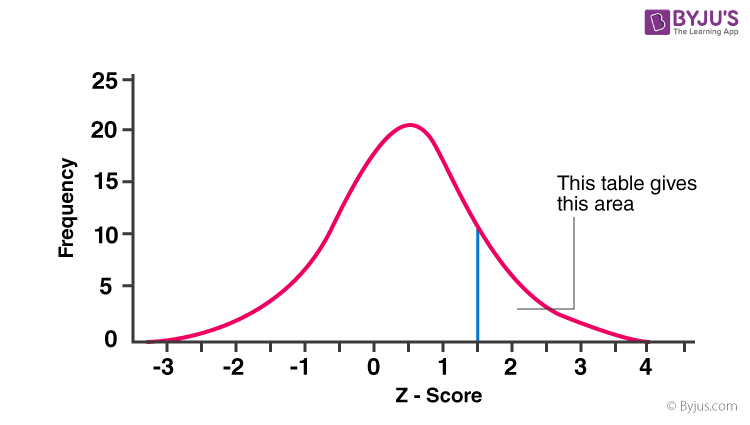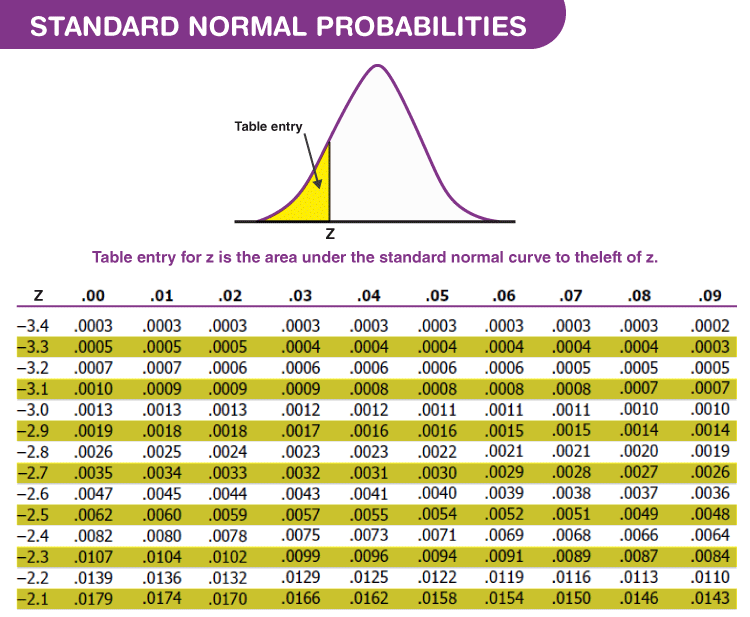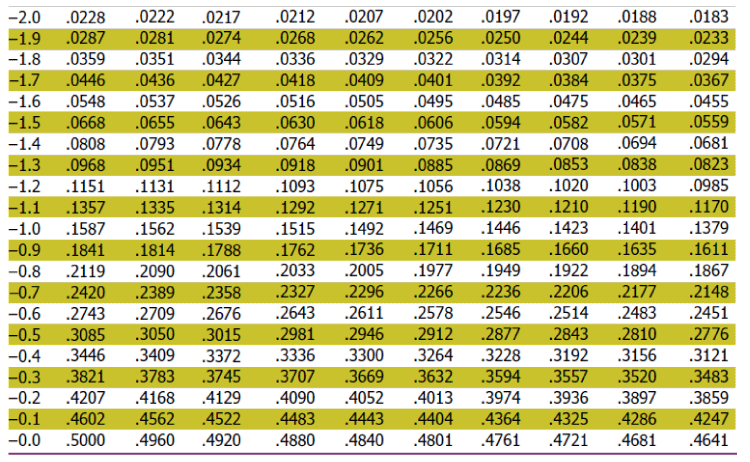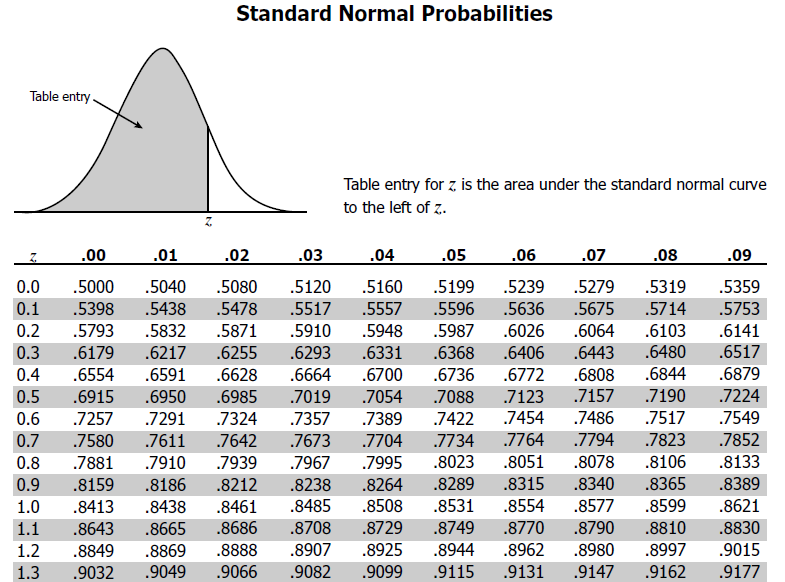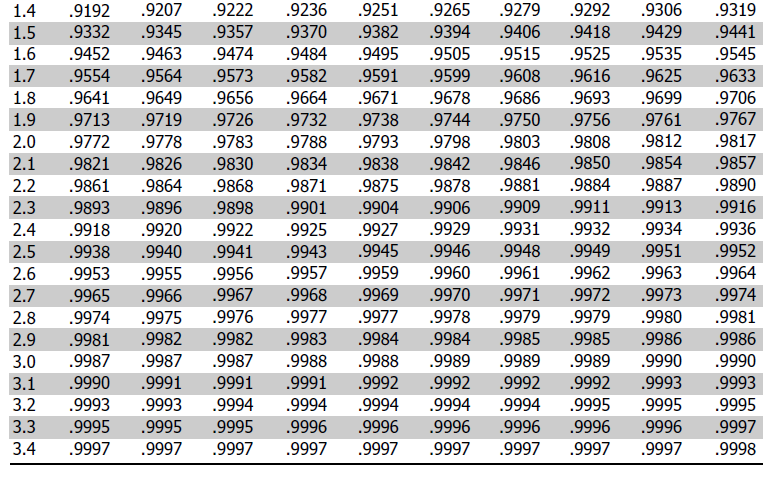## Example of Z Score

Let us understand the concept with the help of a solved example:

Example: The test scores of students in a class test has a mean of 70 and with a standard deviation of 12. What is the probable percentage of students scored more than 85?

Solution: The z score for the given data is,

z= (85-70)/12=1.25

From the z score table, the fraction of the data within this score is 0.8944.

This means 89.44 % of the students are within the test scores of 85 and hence the percentage of students who are above the test scores of 85 = (100-89.44)% = 10.56 %.

Q1

### What does the Z-Score Table Imply?

The z score table helps to know the percentage of values below (to the left) a z-score in a standard normal distribution.

Q2

### What are the Types of Z Score Table?

There are two z-score tables which are:

• Positive Z Score Table: It means that the observed value is above the mean of total values.
• Negative Z Score Table: It means that the observed value is below the mean of total values.
Q3

### What is Z Score and How is it calculated?

A z score is simply defined as the number of standard deviation from the mean. The z-score can be calculated by subtracting mean by test value and dividing it by standard value.

So, z = (x − μ)/ σ

Where x is the test value, μ is the mean and σ is the standard value.

Test your knowledge on Z Score Table